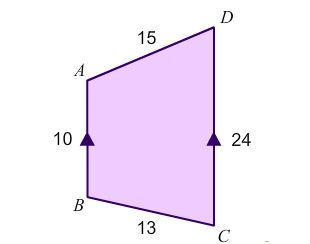# Area of Trapezium? Easy, or is it?

Geometry Level 2$ABCD$ is a quadrilateral with measurements of its sides shown in the above diagram. If $AB$ is parallel to $CD$. What is the area of the quadrilateral?

×